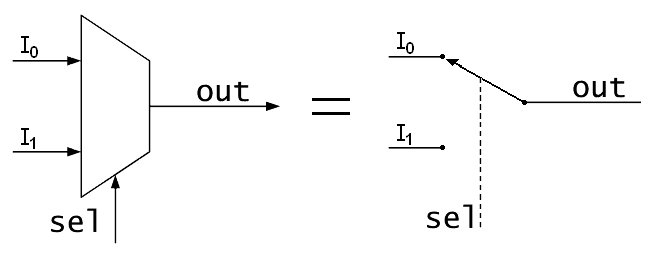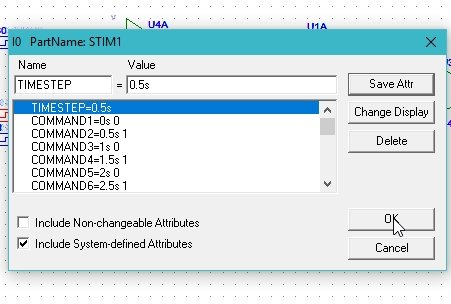# Multiplexer design and simulation using Pspice : tutorial 11

In this tutorial I will explain you the working of a simple multiplexer. At the start a brief and concise introduction to multiplexer specifically a simplest 2×1 multiplexer is provided with the explanation of the output they will show. A multiplexer can also be referred as a mux in short form and 2×1 mux is the simpler of its kind. After that the circuits is simulated using PSPICE and the result are compared with the theoretical discussion provided (which should be same). At the end of the tutorial you are provided with an exercise to do it by yourself, and in the next tutorials I will assume that you have done those exercises and I will not explain the concept regarding them.

### Introduction to multiplexer

A simple multiplexer is used to display a number of input signals on a single output line with the help of a select switch. A total of 2n input signals can be displayed on a single output line with the help of n select switch. A simple multiplexer diagram and functionality is shown in the figure below,Figure 1: Multiplexer

Depending on the value of the select switch one out of all the inputs will be displayed at the output as is obvious from the above figure. To learn the functionality of a multiplexer refer to the simulation example below.

### How to design Multiplexer with PSpice

Figure 2: Opening schematic

Figure 3: Saving schematic

Figure 4: Getting new part

Figure 5: Placing NOT gate

Figure 6: Placing AND gate

Figure 7: Placing OR gate

Figure 8: Placing digital source

Figure 9: Placed components

Figure 10: Drawing wire

Figure 11: Complete circuit diagram

Figure 11: Voltage marker

Figure 1212: Placed voltage marker

Figure 13: Labeling

Figure 14: Naming the device

Figure 15: Input attributes of select line

• The commands in the attributes window represents the value of the input at the corresponding time in the command before the space. The COMMAND1 = 0s 0 shows that at time 0s the value of the input dstm is 0 and the COMMAND2 = 1s 1 shows that the value will update to 1 at time 1 second. Similar is the case with COMMAND5 = 4s 0 that at 4 seconds the value of the input will be 0.
• Next step is to set the attributes of the input digital supply. Double click on the dstm2 supply you connected in the circuit previously and set the input commands of the digital input system but these commands may or may not be same to the commands of input 1 as shown in the figure below,Figure 16: Setting attributes of input 0

Figure 17: Setting attributes of input 1

Figure 18: Simulation setup

Figure 19: Transient response

Figure 20: Simulation

Figure 21: Output

The output of the circuit shows that when the select switch is 0, the value at the input I0 will be displayed at the output Vout. Similarly, when the select switch is 1 then the value at the input line I1 will be mirrored on the output Vout. The select switch will select which out of all the inputs which of the input will be displayed on the single line output.

Exercise:

• Design a 4×1 mux using the same methodology as in case of 2×1 multiplexer (mux)

<<Previous tutorial                                                Next  tutorial>>